Importance: Medium ✭✭
 Author(s): Zhu, Xuding
 Subject: Graph Theory » Coloring » » Vertex coloring
 Keywords: choosability list coloring on-line choosability
 Posted by: Jon Noel on: April 11th, 2013
Question   Are there graphs for which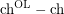is arbitrarily large?

We let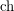denote the (classical) choice number. For a definition of the on-line choice number of(denoted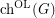), see the following posting: On-Line Ohba's Conjecture.

A result of Alon [Alo93] says that the choice number of a graph is bounded above and below by a function of the colouring number, defined as follows:.

Zhu [Zhu09] demonstrated that the on-line choice number is bounded above by the colouring number. By combining this with Alon's result, we have that there is a function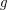such that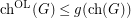for every graph. However, the functionfrom Alon's result is exponential. In [Zhu09], Zhu asked if we can do better (polynomial? linear? etc).

It is known that there are graphs for which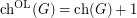. Interestingly, as is mentioned in [CLM+13], it is not even known whether there is a graphsuch that.

There are not many graphs for which the choice number (let alone the on-line choice number) is known exactly. For this reason, it seems that a natural starting point for this problem is to study the complete-partite graph in which every part has size, denoted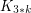. Kierstead [Kie00] proved that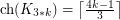. Kozik, Micek and Zhu proved that the.

It may be the case that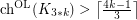. Is it larger than?

## Bibliography

[Alo93] N. Alon. Restricted colorings of graphs. In Surveys in combinatorics, 1993 (Keele), volume 187 of London Math. Soc. Lecture Note Ser., pages 1–33. Cambridge Univ. Press, Cambridge, 1993.

[CLM+13] J. Carraher, S. Loeb, T. Mahoney, G. Puleo, M.-T. Tsai, and D. West. Three Topics in Online List Coloring. Preprint, February 2013.

[HWZ12] P. Huang, T. Wong, and X. Zhu. Application of polynomial method to on-line list colouring of graphs. European J. Combin., 33(5):872–883, 2012.

[Kie00] H. A. Kierstead. On the choosability of complete multipartite graphs with part size three. Discrete Math., 211(1-3):255–259, 2000.

[KKLZ12] S.-J. Kim, Y. S. Kwon, D. D.-F. Liu, and X. Zhu. On-line list colouring of complete multipartite graphs. Electron. J. Combin., 19(1):Paper 41, 13, 2012.

[KMZ12] J. Kozik, P. Micek, and X. Zhu. Towards on-line Ohba’s conjecture. Preprint, arXiv:1111.5458v2, December 2012.

[Sch09] U. Schauz. Mr. Paint and Mrs. Correct. Electron. J. Combin., 16(1):Research Paper 77, 18, 2009.

[Sch10] U. Schauz. A paintability version of the combinatorial Nullstellensatz, and list colorings of k-partite k-uniform hypergraphs. Electron. J. Combin., 17(1):Research Paper 176, 13, 2010.

*[Zhu09] X. Zhu. On-line list colouring of graphs. Electron. J. Combin., 16(1):Research Paper 127, 16, 2009.

* indicates original appearance(s) of problem.